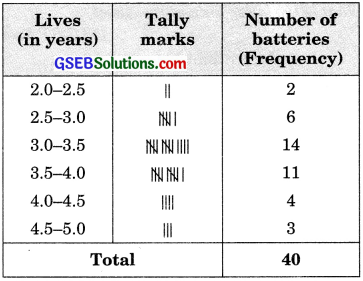# GSEB Solutions Class 9 Maths Chapter 14 Statistics Ex 14.2

Gujarat Board GSEB Solutions Class 9 Maths Chapter 14 Statistics Ex 14.2 Textbook Questions and Answers.

## Gujarat Board Textbook Solutions Class 9 Maths Chapter 14 Statistics Ex 14.2

Question 1.
The blood groups of 30 students of class VIII are recorded as follows:
A, B, O, O, AB, O, A, O, B, A, O, B, A, O, O, A, AB, O, A, A, O, O, AB, B, A, O, B, A, B, O.
Represent this data in the form of a frequency distribution table. Which is the most common and which is the rarest, blood group among these students? [NCERT Exemplar]
Solution: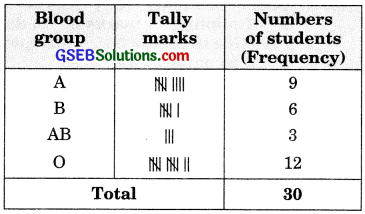O is the most common and AB is the rarest blood group among these students.

Question 2.
The distance (in km) of 40 engineers from their residence to their place of work was found as follows: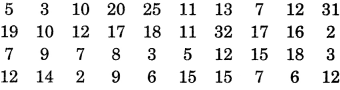Construct a grouped frequency distribution table with class size 5 for the data given above, taking the first interval as 0-5 (5 not included). What main features do you observe from this tabular representation?
Solution: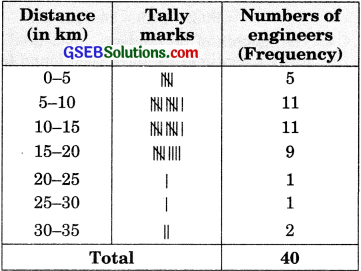We observe the following features from this tabular representation:

1. The distance (in km) from their residence to their work of the maximum number of engineers are less than 20 km.
2. Very few engineers have their residence 20 km or far away from their workplace.

Question 3.
The relative humidity (in %) of a certain city for a month of 30 days was as follows: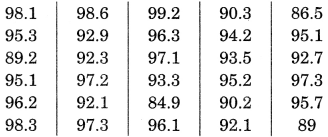(i) Construct a grouped frequency distribution table with classes 84 – 86, 86 – 88 etc.
(ii) Which month or season do you think this data is about?
(ii) What is the range of this data?
Solution: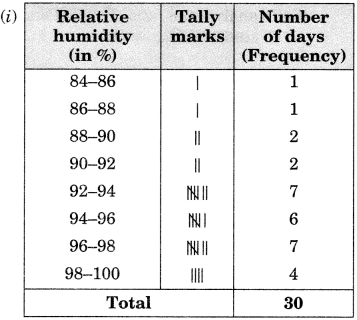(ii) This data appears to be taken in the rainy reason as the relative humidity is high.
(iii) Range = Highest value – Lowest value.
= 99.2 – 84.9 = 14.3 (in %).Question 4.
The heights of 50 students, measured to the nearest centimetres, have been found to be as follows: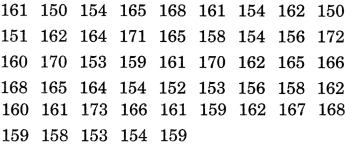(i) Represent the data given above by a grouped frequency distribution table, taking the class intervals as 160-165, 165-170, etc.
(ii) What can you conclude about their heights from the table?
Solution: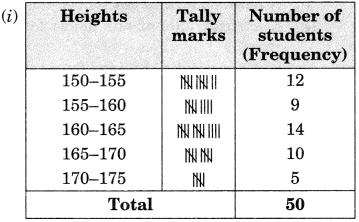(ii) The heights of the maximum number of students are in the group 160-165 and the heights of the minimum number of students are in the group 170-175. More than 50% of students are shorter than 165 cm.

Question 5.
A study was conducted to find out the concentration of sulphur dioxide in the air in parts per million (ppm) of a certain city. The data obtained for 30 days is as follows: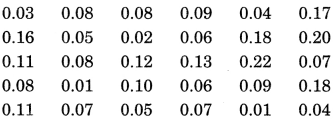(i) Make a grouped frequency distribution table for this data with class intervals as 0.00-0.04, 0.04-0.08, as so on.
(ii) For how many days, was the concentration of sulphur dioxide more than 0.11 parts per million?
Solution: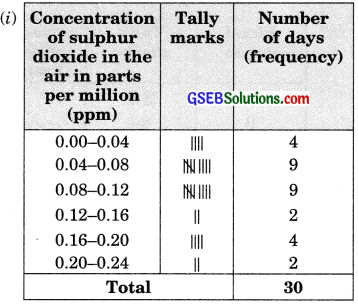(ii) The concentration of sulphur dioxide was more than 0.11 parts per million for 2 + 4 + 2 = 8 days.Question 6.
Three coins were tossed 30 times simultaneously. Each time the number of heads occurring was noted down as follows: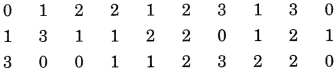Prepare a frequency distribution table for the data given above.
Solution: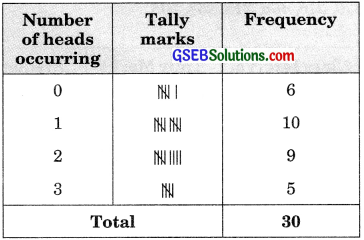Question 7.
The value of it up to 50 decimal places is given below:
3.1415926535897932384626433832795028841 9716939937510.
(i) Make a frequency distribution of the digits from O to 9 after the decimal point.
(ii) What are the most and the least frequency occurring digits?
Solution: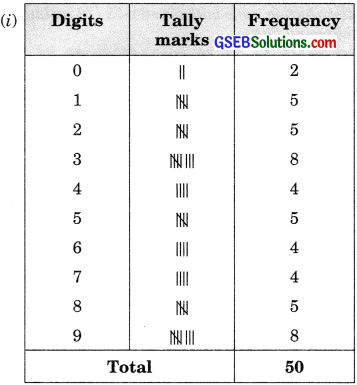(ii) The most frequently occurring digits are 3 and 9. The least frequently occurring digit is O.

Question 8
Thirty children were asked about the number of hours they watched TV programmes in the previous week. The result was found as follows: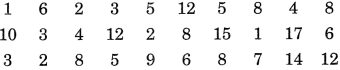(i) Make a grouped frequency distribution table for this data, taking class width 5 and one of the class intervals as 5-10.
(ii) How many children watched television for 15 or more hours a week?
Solution: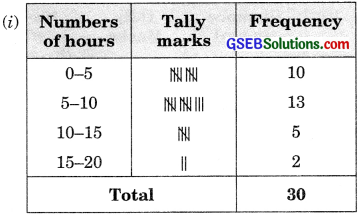(ii) Children watched television for 15 or more hours a week.Question 9.
A company manufactures car batteries of a particular type. The lives (in years) of 40 such batteries were recorded as follows: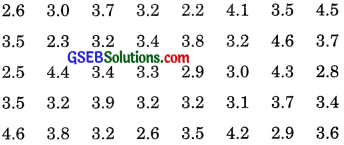Construct a grouped frequency distribution table for this data, using class intervals of size 0.5 starting from the interval 2-2.5.
Solution: